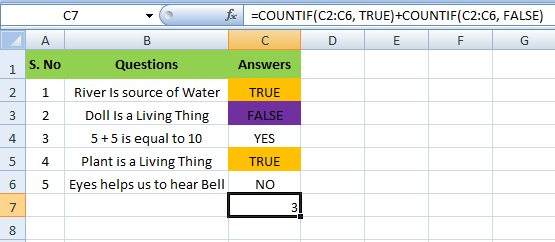### COUNTIF Function In MS Excel

COUNTIF function is used to count cells based on one criteria. It can be used in many ways such as to count the
• Numbers
• Text
• Booleans
Let's learn with Example

### How to Count Numbers using COUNTIF Function

>> COUNTIF function below counts the number of cells that contain the Criteria.
For example, for counting the value 40 , D6 equals =COUNTIF(C2:C6,”40”)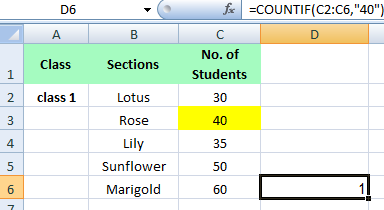>> COUNTIF function below counts the number of cells that are greater than selected Criteria.
For example, for counting the value greater than 40 , D6 equals =COUNTIF(C2:C6,”>40”)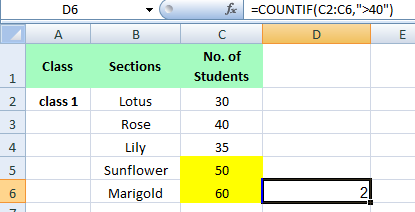>> COUNTIF function below counts the number of cells that are not equal to selected Criteria.
For example, for counting the value not equal to 40 , D6 equals =COUNTIF(C2:C6,”<>40”)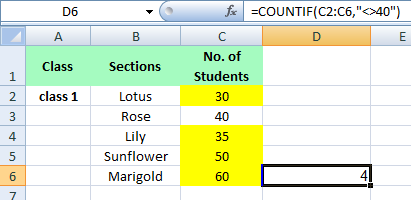>> COUNTIF functions below count the number of cells that are equal to Selected Criteria.
For example, for counting the value equal to 30 Or 40
D6 equals =COUNTIF(C2:C6,30)+COUNTIF(C2:C6,40)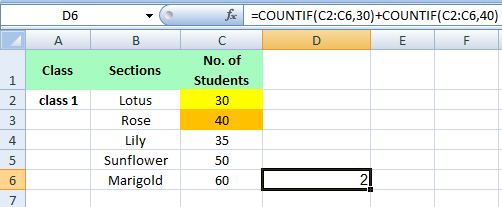### How to Count Text using COUNTIF Function

>> COUNTIF function below counts the number of cells that contains selected Text Criteria.
For example, for counting the Text Red Rose C9 equals =COUNTIF(B2:B9,”Red Rose”)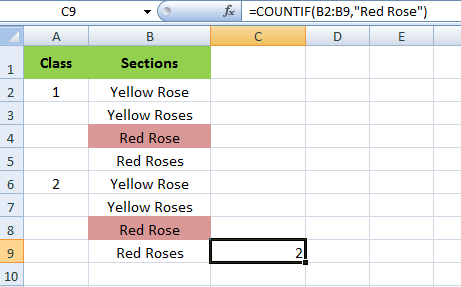>> COUNTIF function below counts the number of cells that contain exactly the selected criteria+1 character.
For example, for counting the Text RedRoses C9 equals =COUNTIF(B2:B9,”RedRose?”)
A question mark (?) matches exactly one character.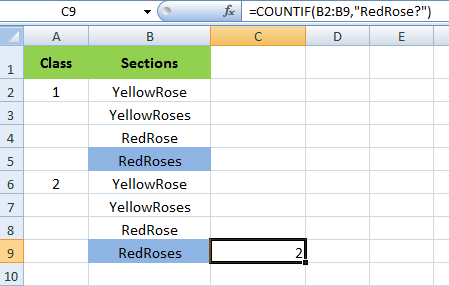>> COUNTIF function below counts the number of cells that contains the Criteria in any way.
For example, for counting the Text Red in any way be it is before, after or with any other text,
C9 equals =COUNTIF(B2:B9,”*Red*”)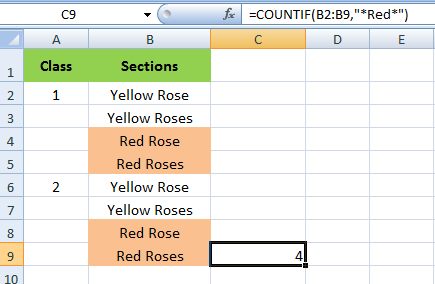### How to Count Booleans using COUNTIF Function

>> COUNTIF function below counts the number of cells that contain the Boolean Criteria.
For example, for counting the Boolean TRUE C7 equals =COUNTIF(C2:C6, ”TRUE”)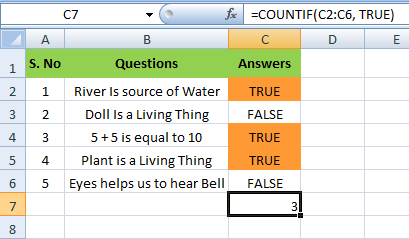Likewise it is done for Boolean, FALSE
For example, for counting the Boolean FALSE C7 equals =COUNTIF(C2:C6,”FALSE”)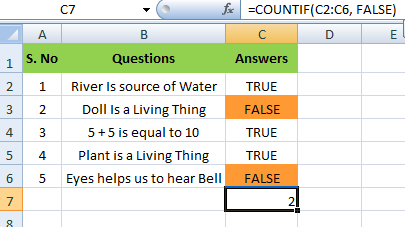>> COUNTIF function below count the number of cells that contain the Either Boolean Criteria
For example, for counting the Boolean TRUE or FALSE ,
C7 equals =COUNTIF(C2:C9,”TRUE”)+COUNTIF(C2:C9,”FALSE”)